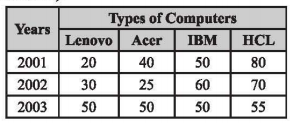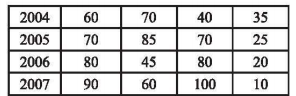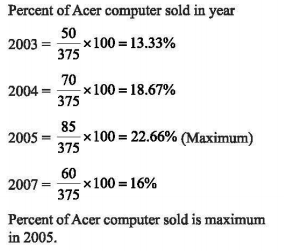# UGC NET Paper 1 December (Pack of 3) - UGC NET PAPER 1 3rd December 2019 Morning Shift

>>>>>>>>UGC NET PAPER 1 3rd December 2019 Morning Shift

The following table gives the sales of computers of different types or brands over the years 2001 to 2007. Based on data, answer the question.
Number of different types of computers sold (In thousands)• A

200%• B

250%• C

300%• D

350%• Option : D
• Explanation : Sales of lenovo computer in 2007 = 90000
Sales of lenovo computer in 2001 = 20000
Increase in sales = 90000 - 20000 = 70,000
Percent increase = 70,000/20,000 X 100 = 350%

The following table gives the sales of computers of different types or brands over the years 2001 to 2007. Based on data, answer the question.
Number of different types of computers sold (In thousands)• A

IBM• B

Lenovo• C

Acer• D

HCL• Option : A
• Explanation : Type of computer sales (in thousands) in all the 7 years
IBM = 50+ 60 + 50+40+70 + 80+ 100 =450
Lenovo = 20 + 30 + 50 + 60+ 70 + 80 + 90 =410
Acer = 40 + 25 + 50+ 70 + 85 +45 + 60 =375
HCL = 80+70 + 55 +35 + 25 + 20+ 10 =295
Hence, maximum sales was for IBM

The following table gives the sales of computers of different types or brands over the years 2001 to 2007. Based on data, answer the question.
Number of different types of computers sold (In thousands)• A

Lenovo• B

Acer• C

IBM• D

HCL• Option : D
• Explanation : For HCL brand sales has increased continuously over the year 2001 to 2007.

The following table gives the sales of computers of different types or brands over the years 2001 to 2007. Based on data, answer the question.
Number of different types of computers sold (In thousands)• A

2003• B

2004• C

2005• D

2007• Option : C
• Explanation :The following table gives the sales of computers of different types or brands over the years 2001 to 2007. Based on data, answer the question.
Number of different types of computers sold (In thousands)• A

15,000• B

20,000• C

45,000• D

60,000• Option : D
• Explanation : Difference in the number of Acer computer sold in 2002 and 2005
= 85,000 - 25,000 = 60,000